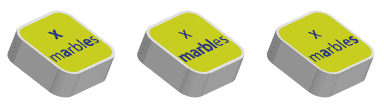#### Example 1

Joe has a pencil case that contains an unknown number of pencils. He has three other pencils. Let x be the number of pencils in the pencil case. Then Joe has x + 3 pencils altogether.#### Example 2

Theresa takes 5 chocolates from a box with a large number of chocolates in it. How many chocolates are left in the box?

#### Solution

Let z be the original number of chocolates in the box.

Theresa takes 5 chocolates so there are z – 5 chocolates left in the box.

#### Example 3

There are n oranges to be divided equally among 5 people. How many oranges does each person get?

#### Solution

Each person receives n ÷ 5 oranges.

#### Example 4

There are three boxes each with the same number of marbles in them. If there are $$x$$ marbles in each box, how many marbles are there in total?#### Solution

There are 3 × x = 3x marbles in total.

#### Summary

The following table gives the meanings of some commonly occurring algebraic expressions.

$$x$$ + 3 The sum of $$x$$ and 33 added to $$x$$, or $$x$$ added to 3 3 more than $$x$$, or $$x$$ more than 3 The difference of $$x$$ and 3, where $$x$$ is greater than or equal to 3 3 is subtracted from $$x$$ 3 less than $$x$$ $$x$$ minus 3 The product of $$x$$ and 3 $$x$$ multiplied by 3, or 3 multiplied by $$x$$ $$x$$ divided by 3 The quotient when $$x$$ is divided by 3 $$x$$ is first multiplied by 2 and then 3 is subtracted $$x$$ is first divided by 3 and then 2 is subtracted

#### Expressions with zeros and ones

Zeros and ones can often be eliminated. For example:

$$x + 0 = x$$ (Adding zero does not change the number.)

$$x × 1 = x$$ (Multiplying by 1 does not change the number.)